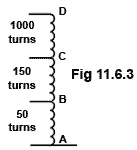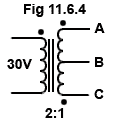# Transformers Quiz

Try our quiz, based on the information you can find in Module 11. Submit your answers and see how many you get right. If you get answers wrong, just follow the hints on the answers page to find the right answer and learn more about transformers as you go.

### 1.What is the primary voltage applied to the transformer illustrated in Fig 11.6.1?

### 2.What is the value of current flowing through the resistor R in Fig 11.6.2?

### 3.

Which solution from the following may be used to overcome hysteresis losses in power transformers?

### 4.

Flux linkage between primary and secondary windings of a transformer is proportional to which of the following?

### 5.Refer to the diagram of an autotransformer in Fig. 11.6.3.
If the voltage across A and D is 230V, what will be (approximately) the voltage across A and B?

### 6.

What will be the approximate turns ratio of a transformer matching a microphone of 60Ω impedance to the 47kΩ input impedance of an amplifier?

### 7.

Which of the following would be the most important property of the material, used for an electromagnetic shield around a RF transformer?

### 8.Refer to the diagram of a transformer having a continuously wound, centre tapped secondary winding in Fig. 11.6.4:
What is the relationship between voltages across AB and BC when B is used as the common terminal?

### 9.

Refer to Fig 11.6.4:
What is the voltage between A and C when B is connected to 0V?

### 10.

How are eddy current losses reduced in the cores of RF transformers?